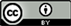1烟台大学，机电汽车工程学院，山东 烟台

2山东上汽汽车变速器有限公司，山东 烟台1. 引言

2. 鼓形齿的鼓形设计

R c 2 = b c 2 + ( R c − C c ) 2 = b c 2 + R c 2 − 2 R c ⋅ C c + C c 2 (1)

R c = b c 2 2 C c .(2)

R c i = b c i 2 2 C c i . (3)

C c i = C c ( b c i b c ) 2 . (4)

f H β 1 = 0

f H β 2 = f H β max ⋅ 1.6875 × 2 1.6875 × 8 = 0.014 × 0.25 = 0.0035

f H β 3 = f H β max ⋅ 1.6875 × 4 1.6875 × 8 = 0.014 × 0.5 = 0.007

f H β 4 = f H β max ⋅ 1.6875 × 6 1.6875 × 8 = 0.014 × 0.75 = 0.0105

f H β 5 = f H β max ⋅ 1.6875 × 7 1.6875 × 8 = 0.014 × 0.875 = 0.01225

C b 3 = 0.01273 − f H β 3 = 0.01273 − 0.007 = 0.00573

C b 2 = 0.00774 − f H β 2 = 0.00774 − 0.0035 = 0.00424

C b 4 = 0.01489 − f H β 4 = 0.01489 − 0.0105 = 0.00423

C b 5 = 0.01508 − f H β 5 = 0.01491 − 0.01225 = 0.00266

R c 2 = b c 2 2 2 C c 2 = ( 1.6875 × 2 ) 2 2 ( 0.00573 − 0.00424 ) = 3822.4   mm

R c 3 = b c 2 2 C c 3 = ( 1.6875 × 4 ) 2 2 × 0.00573 = 3975.8   mm

R c 4 = b c 4 2 2 C c 4 = ( 1.6875 × 2 ) 2 2 ( 0.00573 − 0.00433 ) = 4024.8   mm

R c 5 = b c 5 2 2 C c 5 = ( 1.6875 × 3 ) 2 2 ( 0.00573 − 0.00266 ) = 4174.1   mm

δ 1 = R c 3 − R c 2 R c 3 × 100 % = 3978.5 − 3822.4 3978.5 × 100 % = 3.92 %

δ 2 = R c 4 − R c 3 R c 4 × 100 % = 4024.8 − 3975.8 4024.8 × 100 % = 1.22 %

δ 3 = R c 5 − R c 3 R c 5 × 100 % = 4174.1 − 3975.8 4174.1 × 100 % = 4.75 %

3. ANSYS应力仿真来验证

4. 结束语